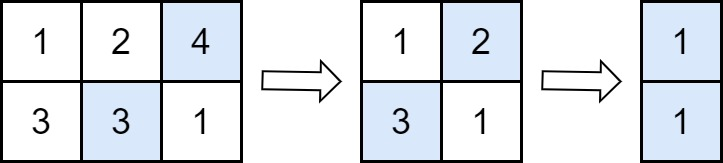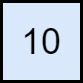2500. Delete Greatest Value in Each Row

#### QUESTION:

You are given an m x n matrix grid consisting of positive integers.

Perform the following operation until grid becomes empty:

Delete the element with the greatest value from each row. If multiple such elements exist, delete any of them. Add the maximum of deleted elements to the answer. Note that the number of columns decreases by one after each operation.

Return the answer after performing the operations described above.

Example 1:``````Input: grid = [[1,2,4],[3,3,1]]
Output: 8
Explanation: The diagram above shows the removed values in each step.
- In the first operation, we remove 4 from the first row and 3 from the second row (notice that, there are two cells with value 3 and we can remove any of them). We add 4 to the answer.
- In the second operation, we remove 2 from the first row and 3 from the second row. We add 3 to the answer.
- In the third operation, we remove 1 from the first row and 1 from the second row. We add 1 to the answer.
The final answer = 4 + 3 + 1 = 8.
``````

Example 2:``````Input: grid = []
Output: 10
Explanation: The diagram above shows the removed values in each step.
- In the first operation, we remove 10 from the first row. We add 10 to the answer.
``````

Constraints:

``````m == grid.length
n == grid[i].length
1 <= m, n <= 50
1 <= grid[i][j] <= 100
``````

#### EXPLANATION:

1. 首先进行排序
2. 找出每列最大的数,加resut上
3. 返回result

#### SOLUTION:

``````class Solution {
func deleteGreatestValue(_ grid: [[Int]]) -> Int {
var grid = grid
var result = 0
for index in grid.indices {
grid[index] = grid[index].sorted(by: >)
}
for indexI in 0..<grid.count {
var tmpResult = 0
for indexJ in 0..<grid.count {
tmpResult = max(tmpResult, grid[indexJ][indexI])
}
result += tmpResult
}
return result
}
}
``````